# Category Archives: Tips and Techniques

Tips, tricks, and how-tos on working with Tableau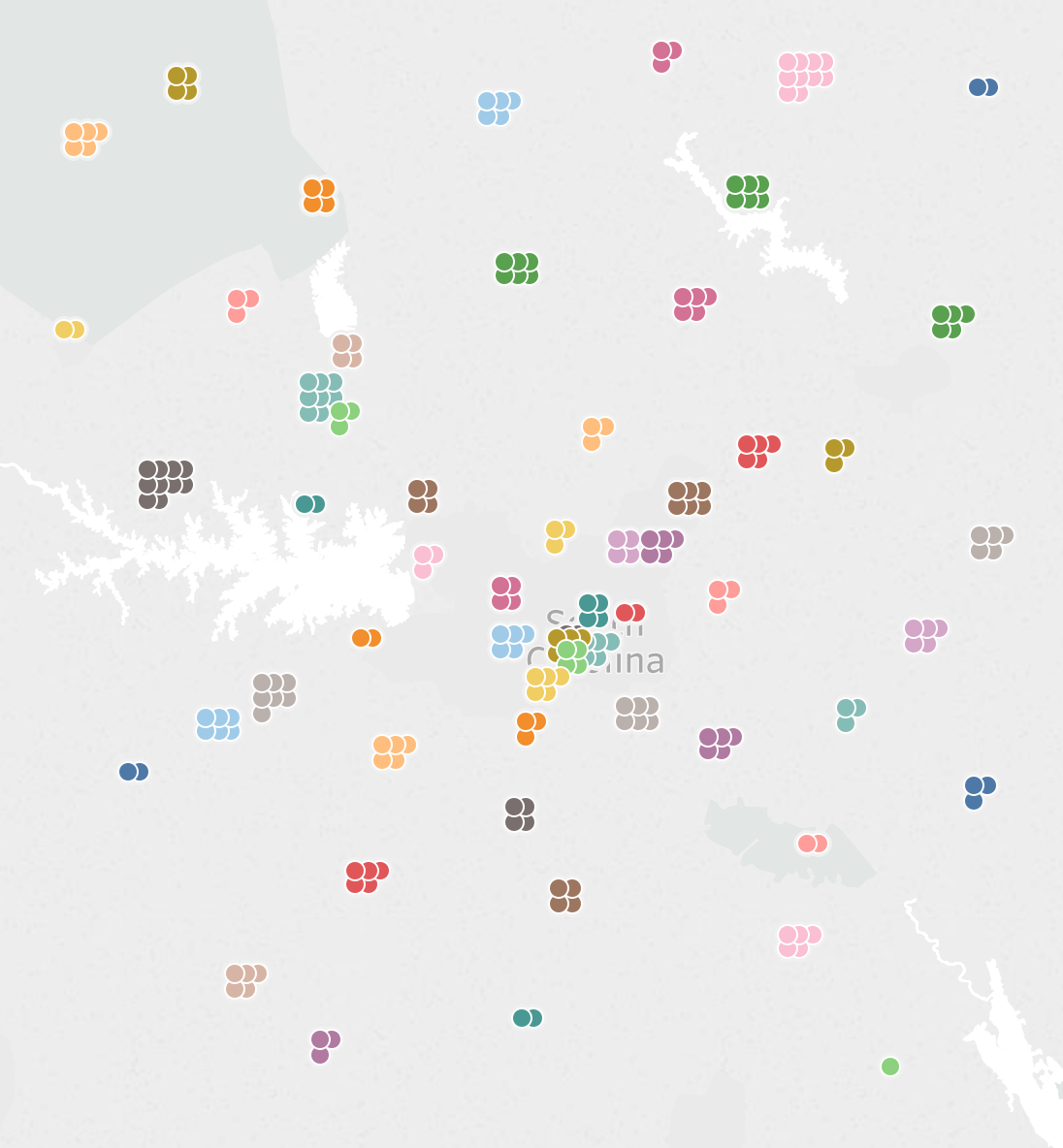# Waffle Charts and Unit Charts on Maps

One of the people I really wanted to meet at #data17 was Sarah Battersby, if you haven’t seen her blogs on mapping you’re missing out. It turns out she wanted to meet me, too!

So Sarah does really awesome stuff (can you tell I’m a fanboy yet??) and yesterday she posted about doing spiral jittering on maps:That inspired me to dig out an old post where I’d done a waffle chart or unit chart jittering technique on a time series: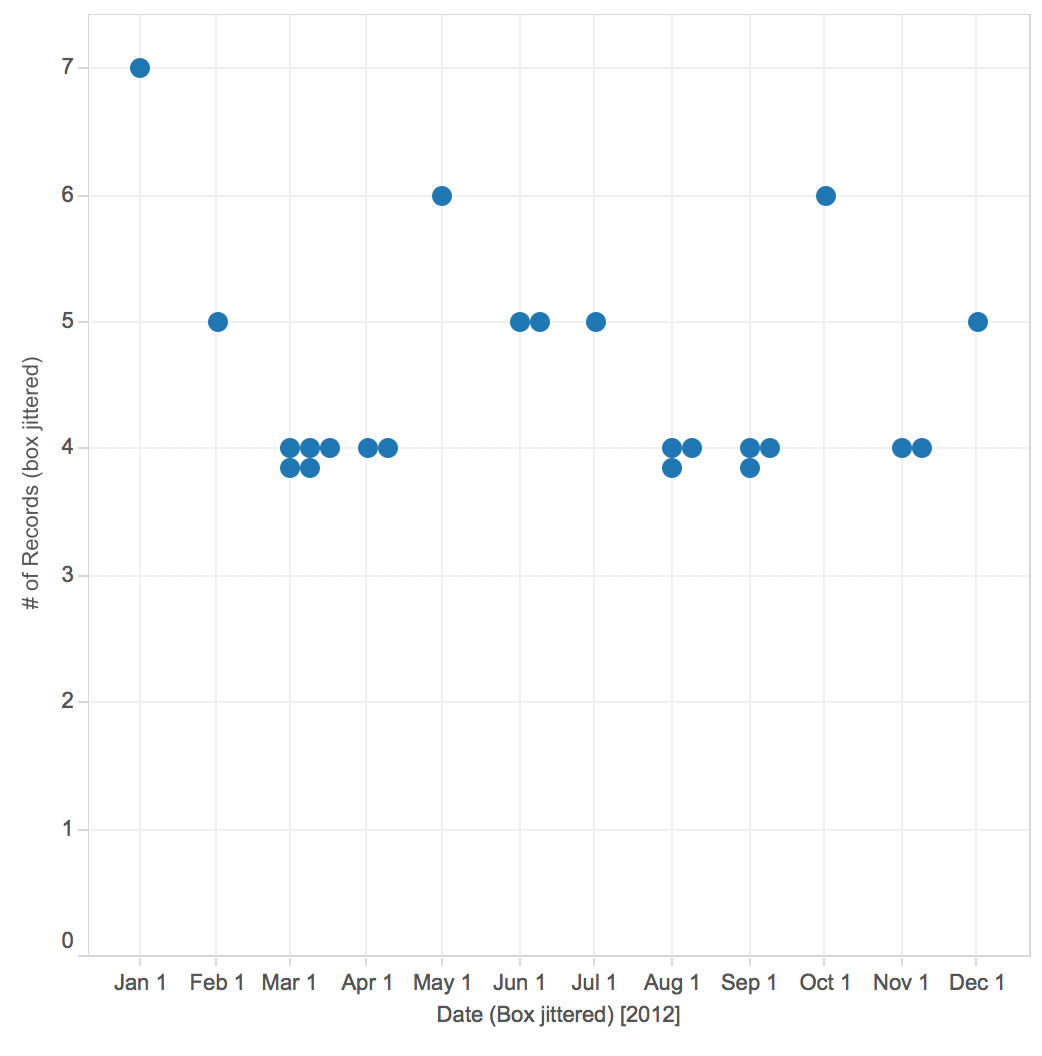And adapt that to a map, like this one that makes me think of bunches of grapes:# Why would we need to do this?

I’m not going to go into all the details, you can go read Sarah’s fantastic Jittering post instead for an overview. Basically there are times when we have more than one “thing” for one or more point(s) on a map and we want to show them that way. For example in the map below there are 5 IDs for the selected zip code that are all plotted on top of one another. Through using jittering we can show a mark for each ID like in the jittered map above where that one visible circle is replaced by five circles.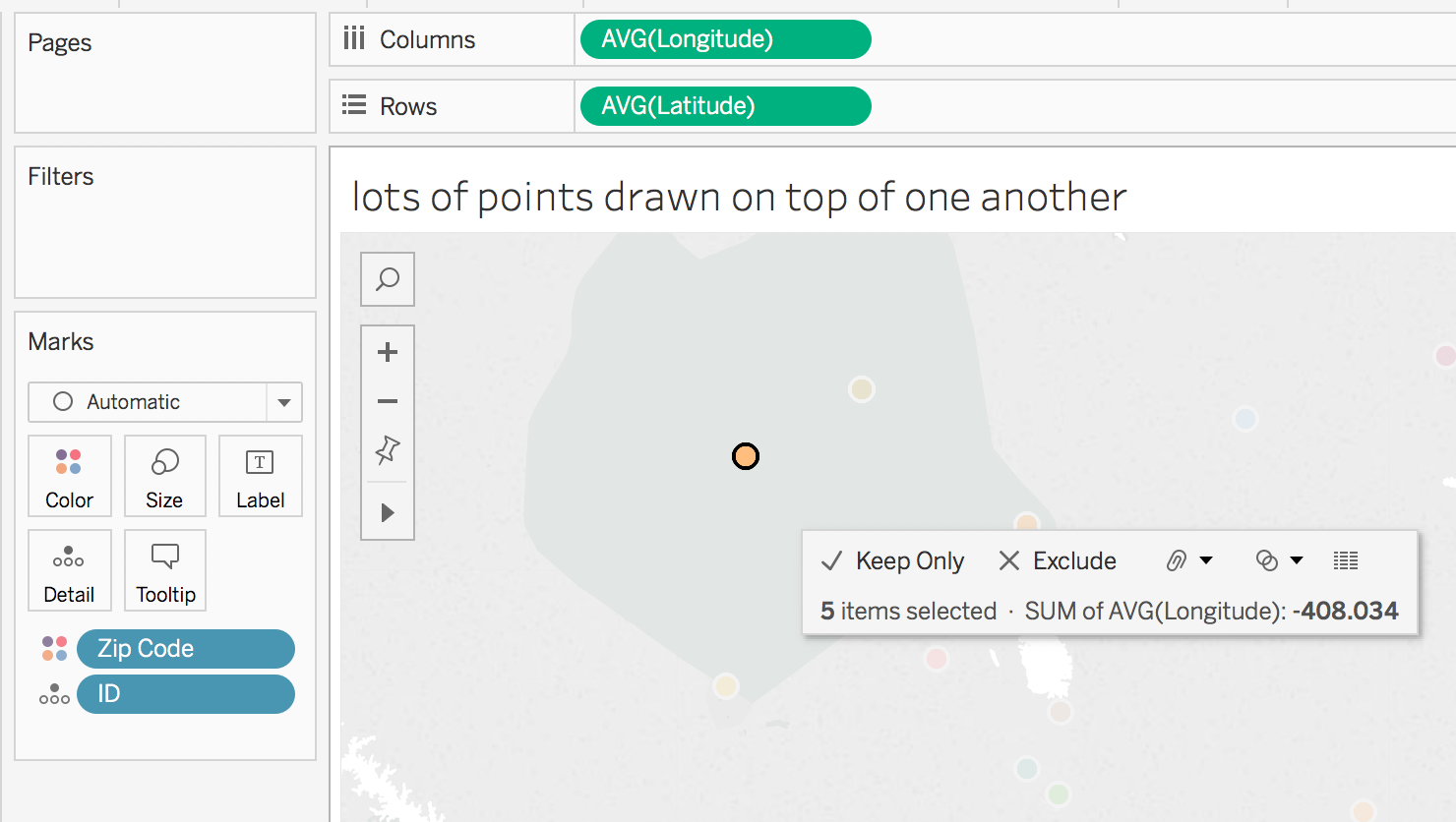# There is nothing new under the sun

Back in 2013 Stephen Few proposed the idea of bricks on maps as a way of encoding a value, the technique I’m using here could be used to get the same result. The key difference is that instead of using the unit or waffle chart to encode the single value we’re using the unit chart to encode multiple values. Andy Cotgreave also had a nice post on some of the issues with this kind of chart.

# How do we put a waffle chart on a map?

First of all we need a data source that has latitudes & longitudes that we can do calculations on. If you don’t there are a variety of ways to get one. After that we need to get mathy. Here’s my workout view: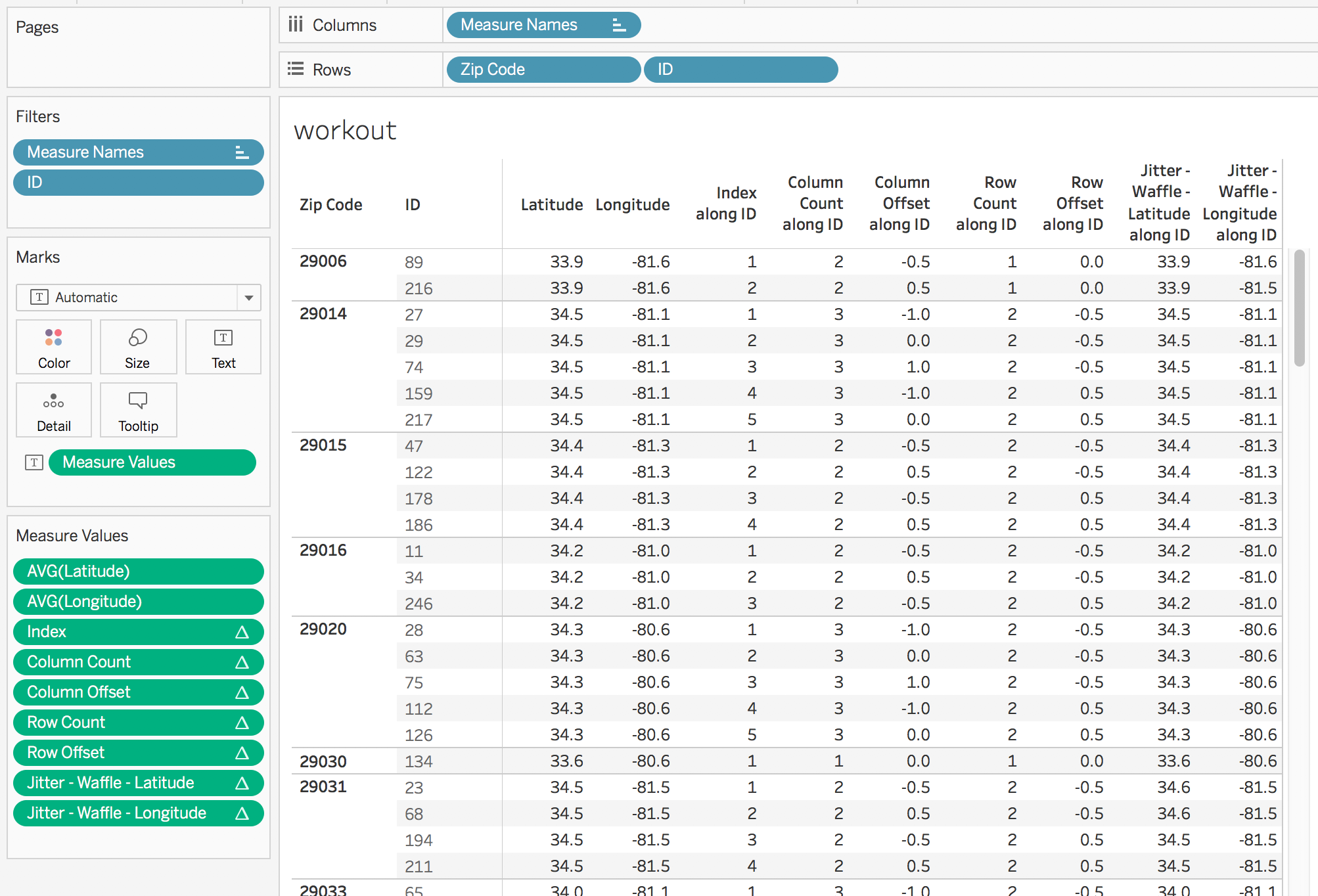All of the table calculations have a compute using on ID, the idea is that the compute using is set to the dimension(s) that are making the extra marks and then the dimension(s) left for partitioning (Zip Code in this case) are the ones that are creating the initial map positions.

I’ll briefly walk through each calculation.

Index uses Tableau’s INDEX() formula and uniquely identifies each mark.

Column Count gets us a count of columns that is based on forming the smallest possible square that will contain all the marks, here’s the formula:

```//this formula will try to draw a square, if you want something else then just put in a fixed aggregate number like MIN(3)
IF INT(SQRT(SIZE())) == SQRT(SIZE()) THEN
SQRT(SIZE())
ELSE
INT(SQRT(SIZE()))+1
END```
This would be a lot simpler if Tableau supported the CEILING() function for table calculations, because then it would be CEILING(SQRT(SIZE())). Vote up https://community.tableau.com/ideas/6239 if you’d like that too.

Column Offset then computes the number of positions of lateral (latitude) offset for each mark from the center of the unit chart. I’ve done some different experiments on where to position the unit chart and for me the most natural is to have the center of the unit chart be the centroid of the point.

```//this gets the column position in the row
IF [Index] % [Column Count] = 0 THEN
[Column Count]
ELSE
[Index] % [Column Count]
END
//subtract the position of the midline to get the offset
- ([Column Count] + 1)/2```

Row Count then gets the number of rows in the unit chart, and would again benefit from a CEILING() function for table calcs (hint hint).

```IF INT(SIZE()/[Column Count]) = SIZE()/[Column Count] THEN
SIZE()/[Column Count]
ELSE
INT(SIZE()/[Column Count]) + 1
END```

Row Offset computes the number of positions of vertical (longitude) offset for each mark from the center of the unit chart:

```//row position in column
IF INT([Index]/[Column Count]) = [Index]/[Column Count] THEN
[Index]/[Column Count]
ELSE
INT([Index]/[Column Count]) + 1
END
//subtract the midline of the row to get the offset
- ([Row Count] + 1)/2```

Jitter – Waffle – Latitude then gets the latitude for the point and subtracts the row offset multiplied by a waffle jitter parameter that lets us deal with the zoom level to offset by just the right amount of latitude. This field has the Latitude geographic role assigned.

`TOTAL(AVG([Latitude])) - [Row Offset] * [waffle jitter]`

Jitter – Waffle – Longitude does the same for the longitude.

`TOTAL(AVG([Longitude])) + [Column Offset] * [waffle jitter]`

Note that I’ve only tested this so far with US latitude & longitudes, these calcs might need to be slightly different if you are east of 0 longitude or south of the equator.

# Building the View

With all the calcs created in the workout sheet and verified, then we can duplicate that sheet and start dragging & dropping pills to build a view. In this case I’ve created a dual axis on to show that the centers of the unit chart are on the centroids of the zip codes (the black crosses) and at this zoom level a waffle jitter value of 0.02 works well: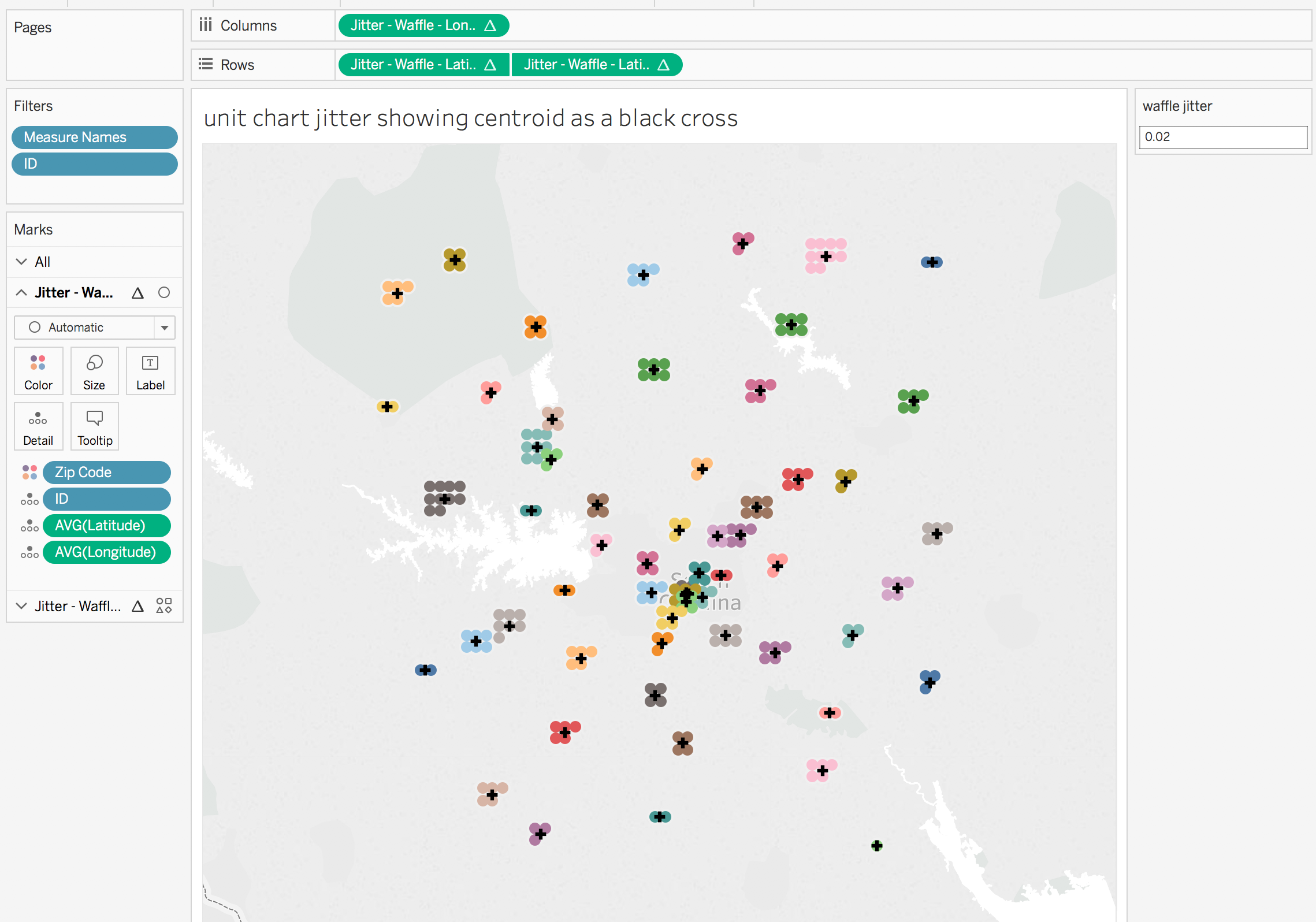For this view I’ve made the waffle jitter slightly smaller to 0.015 degrees and added white borders to the marks:# Why Stop Here?

We don’t have to use just circle marks, in this view I’ve used shape marks and different colors for each shape just to show how much information we could place on a single view.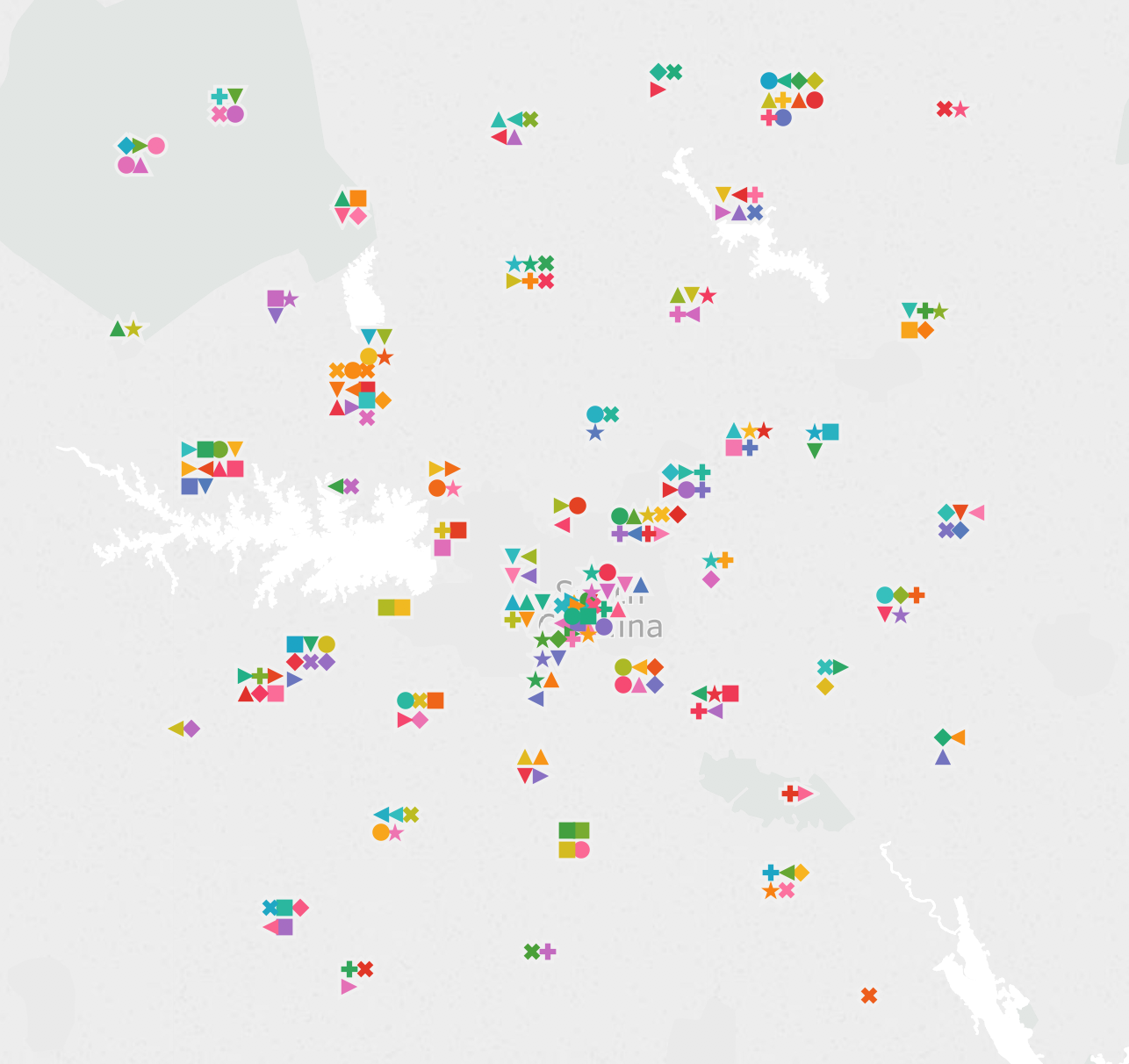And in Tableau v10.5 we could even do something like only show the unit chart on a map if there weren’t too many marks nearby (by using a binning or hex bin function in an LOD expression) and use the new Viz in Tooltip feature to get the detail.

# Conclusion

Thanks to Alan Eldridge for the original jittering post and Sarah for adding more jittering options and inspiring this post! Here’s the unit or waffle chart jitter on maps workbook on Tableau Public.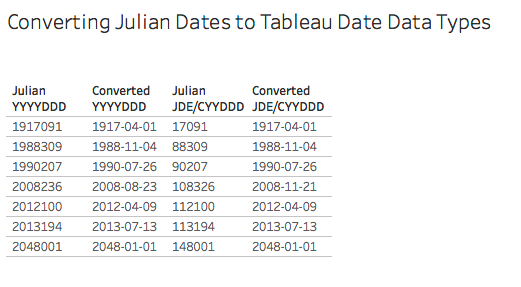# A Dating Lesson: Using Math to Get Dates (by Converting Julian Dates)

I recently had to convert some date fields formatted as Julian dates into more usable Tableau date data types (it’s not a date format that Tableau natively understands) and my web search got me a little frustrated because all the solutions I found were long formulas using string manipulation functions like LEFT(), RIGHT(), etc. That kind of solution can get the job done, but there’s something to be left desired:

String manipulation is sloooooooooooooooooooooooooooooowwww.

How slow? How about one thousand times slower (or more) than using math functions! I like my calculations to be efficient from the beginning: The practical reason is that I don’t have to spend time later going back to do performance tuning. Computers were built to do math really really fast so whenever possible I’ll use math to do my date conversions. The aesthetic reason is that there’s an elegance to creating equations that use the least number of steps, and the play reason is because math is fun!

Therefore in this post I’ll describe a faster, more elegant, and more fun way (IMO) of converting Julian dates into Tableau dates. Note that in some cases you might be able to do the conversion to a date data type inside your database, that could be even faster than the formulas I describe here.

## What is a Julian date, anyways?

There are two formats of Julian dates that I’ve run into:

• The JDE (for JD Edwards) or CYYDDD format where the DDD is the day of the year (a number from 1 to 366), YY is the two digit year, and C is the century where 0 is 1900, 1 is 2000, and so on. For example 5001 is 1905-01-01 and 117365 is 2017-12-31.
• The “modern Julian date” or YYYYDDD format where DDD is again the day of year and YYYY is the four digit year, so 2017365 would be 2017-12-31.

Now the Julian dates might be stored in raw data as strings or as numbers, either way we’re going to work with them as numbers. So if you’re starting out with Julian dates as strings then the first step is to right-click on those fields and change the data type to Number (Whole).

## Learning Three Math & Date Techniques

We can convert Julian JDE/CYYDDD dates in a single formula:

```DATE(DATEADD('day',[Julian JDE/CYYDDD] % 1000 - 1,

There are three techniques at work in this formula:

1. Extract the right-most N digits from a number using the modulo operator, for example to get the DDD value.
2. Remove the right-most N digits from a number to extract the left-most M digits from a number using the INT() function, for example to get the CYY value.
3. Using DATEADD() to add intervals to a starting date and nest for each date_part as necessary.

### Extracting the right-most N digits from a number

The slow way to get the right-most N digits from a number would be something like INT(RIGHT(STR([number]),N)). We can completely avoid the string conversion and need for RIGHT() by using some math.

The modulo operator % is a math function that returns the remainder for a whole number. So 5 % 2 returns 1, 17 % 10 returns 7, and so on. We can use this to return the right-most N digits from a number without needing to do any string manipulation by using [number] % 10^N.

In our case `[Julian JDE/CYYDDD] % 1000` will return the last 3 digits of the field corresponding to the DDD aka day of year.

A couple of other uses of the modulo function are jittering marks in a dot plot and creating groups/bins based on the right-most digits when numbers are categorical variables.

### Remove the right-most N digits from a number to extract the left-most M digits from the number

The starting way to get the left-most M digits from a number would be something like INT(LEFT(STR([number]),M)). However this gets more complicated with the JDE/CYYDDD format because depending on the date then we might want the 2 left most digits (for years in the 1900s) or the 3 left most digits (for years in the 2000s) which would lead to even more string manipulation to get the desired result, for example here’s a formula I found online for getting the year: `IF LEN([Julian JDE/CYYDDD]) = 6 AND LEFT([Julian JDE/CYYDDD], 1) = '1' THEN '20' + MID([Julian JDE/CYYDDD], 2, 2) ELSEIF LEN([Julian JDE/CYYDDD]) = 5 THEN '19' + MID([Julian JDE/CYYDDD], 1, 2) END`. All those LEN(), LEFT(), and MID() calls will be slooooww.

Thinking about this from a math standpoint what we want to do is with the JDE/CYYDDD format is to remove the DDD (the right-most N digits) and extract what’s left. The way we can do that is in two steps: 1) turn the DDD portion into a decimal so CYYDDD becomes CYY.DDD, then 2) truncate the DDD decimal portion so we’re just left with the CYY.

We can do that with the formula INT([number] / 10^N). The [number] / 10^N divides the number by the number of digits we want to remove, so 5001 becomes 5.001, 117365 becomes 117.365, and so on. Then the INT() around that truncates the decimal places and leaves us with the remaining whole number.

In our case` INT([Julian JDE/CYYDDD] / 1000)` removes the right-most three digits so what we’re left with is the century and number of years.

I regularly use this technique to build my own bins, for example INT([Sales] / 100) * 100 creates bins of 0, 100, 200, and so on.

### Using DATEADD() to add a number of intervals to a starting date

Tableau’s DATEADD() function takes three arguments:

• date_part: a value like ‘day’, ‘month’, ‘hour’, etc.
• interval: a whole number of intervals
• date: a starting date

The advantage of using DATEADD() over something like DATE([lots of string manipulation to build a string of YYYY-MM-DD or MM/DD/YYYY or DD/MM/YYYY format]) is again that we’re avoiding all that string manipulation and just doing date math, which is really math.

With the first two techniques we’ve converted the JDE/CYYDDD format to into the intervals for the day date_part and the intervals for the year date_part, so now we can use DATEADD() once for each date_part in a nested fashion. All we need is a starting date, and in this case we can take advantage of the CYY structure to figure out the appropriate starting date. This is the number of years since 1900, so to generate the year we can use:

`DATEADD('year',INT([Julian JDE/CYYDDD]/1000),#1900-01-01#)`

Then to add the DDD day of year we can use DATEADD() with a starting date of the year date we just made. We do need to subtract 1 from the DDD day of year because the first day of the year is 1, not 0.

```DATEADD('day',[Julian JDE/CYYDDD] % 1000 - 1,

## Julian JDE/CYYDDD Conversion

Tableau’s DATEADD() always returns a datetime data type, so using the above formula and wrapping the calculation in a final DATE() gives us the desired date data type:

```DATE(DATEADD('day',[Julian JDE/CYYDDD] % 1000 - 1,

Here’s a Tableau view showing the DDD and CYY calculations along with the final Converted JDE/CYYYDDD calculation: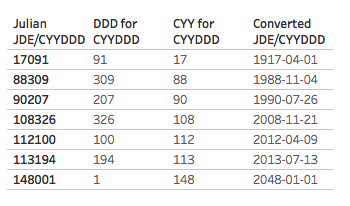## Julian YYYYDDD Conversion

The YYYYDDD conversion uses a quite similar formula, with one change:

```DATE(DATEADD('day',[Julian YYYYDDD] % 1000 - 1,

The difference is that the YYYY portion of the calculation is returning the full four digit year so we subtract 1900 from that to get a number of years to add to the 1900-01-01 start date.

Here’s a Tableau view showing the DDD and YYYY calculations along with the final Converted YYYYDDD calculation: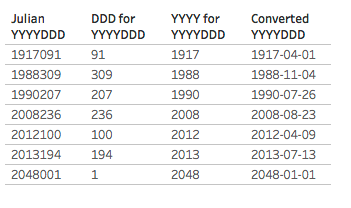## Conclusion

Math is fun. Math is fast. Math is your friend. Use math to get dates. (Date data types, that is).

Here’s a link to the converting julian dates to dates workbook on Tableau Public.

A little plug: If you like this post (and can tolerate the occasional bad pun) and want help in finding dates (in your data) please check out my company, DataBlick. We offer Tableau and Alteryx support, consulting, and training and can help you get the most out of your data!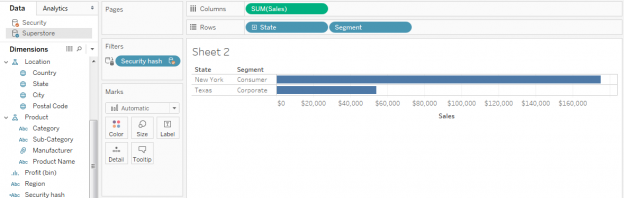# Row Level Security Using Tableau 10.0 Cross Data Source Filters by Jamieson Christian

Jonathan here. Serendipity is a wonderful thing! I was briefly on the Tableau community forums last week and found this thread where Jamieson Christian described a new method for user- row-level security in Tableau that I hadn’t seen before, and it’s so awesomely cool I asked him if he could blog about it, and he accepted my invitation! I’ve already implemented this method at a client and it brought a dashboard from unacceptable refresh times to nearly instantaneous. So read on for Jamieson’s description.

Row level security often presents challenges, if your security data is not in the same data source as your main data. In such cases, solutions tend to leverage one of four techniques:

1. Data prep outside of Tableau – often involves cross products that can make data sets unacceptably large.
2. Passing parameter-based filter through Tableau’s Javascript API – requires using a portal that is not always feasible for organizations, also won’t work with Tableau Mobile.
3. Data blending — just about the only in-Tableau option prior to Tableau 10.0.
4. Cross data source joins — available in Tableau as of Tableau 10.0.

This post introduces a 5th option using a cross data source filter. see below for how to set it up!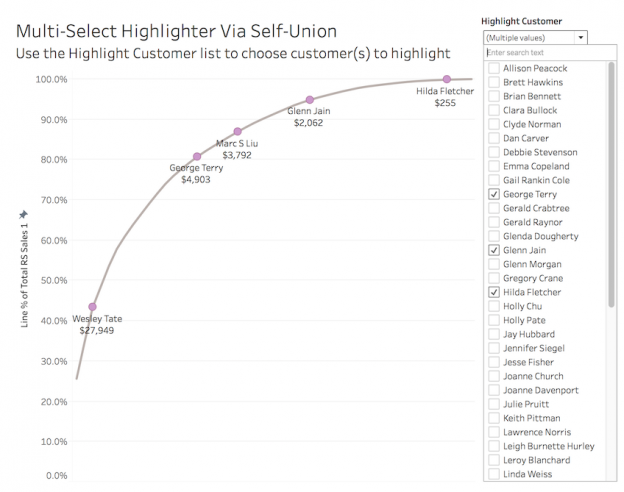# Multiple Ways to Multi-Select and Highlight in Tableau

Last year in version 10.0 Tableau introduced the highlighter that lets us quickly highlight marks. It’s got two potential limitations, though:

1. We can only highlight a single value or all marks meeting a search criteria, not multi-select values.
2. Tableau’s Highlighter (and Highlight Actions) are hard-coded so we don’t have control over the formatting of the highlighted marks.

We can work around those limits in a few different ways:

• Using a separate worksheet with Highlight Action(s) enabled with Hover or Select.
• Using a separate worksheet with Highlight Action(s) enabled, a dual axis, and a duplicated dimension to display different marks. This technique was developed by Rody Zakovich in his post Only Color Marks on Dashboard Highlight .
• Using a self-union’ed data source and a dual axis to get total control over how the highlighted marks are displayed and have more control over the user interface for choosing the highlighted marks. I think I might have invented this technique, I haven’t seen anything quite like it before. This method lets us build views like this: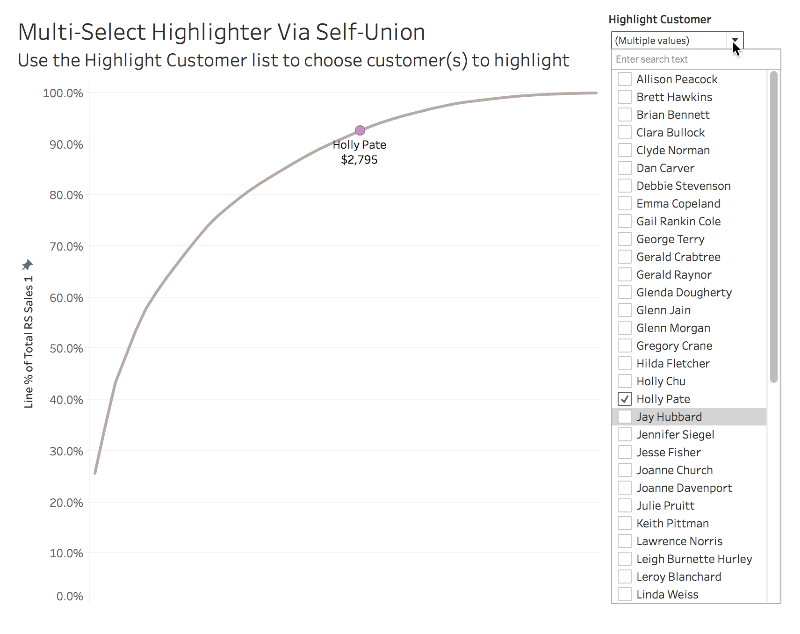Read on for how to build these out and choose the right method for you!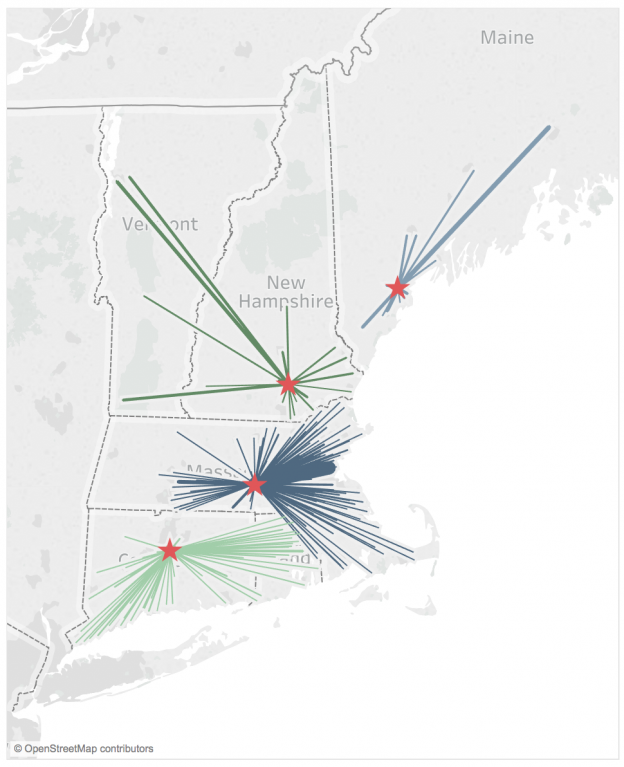# Cross Data Source Joins + Join on Calcs = UNION Almost Anything in Tableau v10.2

Since Tableau v9.0 or so every new release has come with new features that simplify and reduce the amount of data prep I have to do outside of Tableau. Pivot in version 9.0, the first batch of union support in v9.3, support for ad hoc groups in calculations, cross data source joins and filters in v10.0, and more in-database unions and join calculations in v10.2. With the join calculations we can now do unions and cross/cartesian joins within or across almost any data source without needing Custom SQL or linked databases and without waiting for Tableau to implement more union support, read on to learn how!

Here are some use cases for unions across data sources:

• Union data that is coming from different systems, for example when different subsidiaries of an organization are using different databases but you want a single view of the company.
• Union actual sales data from transactional systems and budget data that might come from an Excel spreadsheet.
• Union customer & store/facility data sets so you can draw both on the same map.

This post goes through examples of all three using a combination of text files and superstore, and Rody Zakovich will be doing a post sometime soon on unions and joins with Tableau data extracts. (Did you know you could do cross data source joins to extracts? That capability came with v10.0, and we can have all sorts of fun with that using join calculations!)